### Home > CALC > Chapter 3 > Lesson 3.3.1 > Problem3-88

3-88.
1. Given f(x) below, find f ′(x). Homework Help ✎

1. f(x) = −x

2. f(x) = 0

3.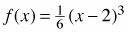4. f(x) = 9x + sin x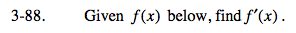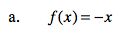You should know by now that a derivative is a slope function. Notice that this is a linear equation, and linear equations have constant slopes.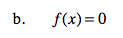SImilarly, this is a horizontal line. What is the slope of a horizontal line? That will also be the derivative.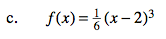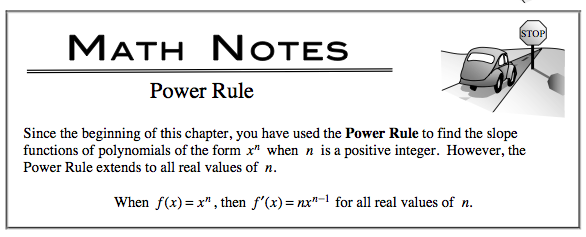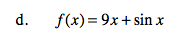Recall that the derivative of sin(x) is cos(x).